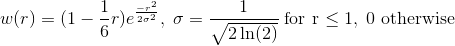Observations influence in the model error subspace can be decreased using decorrelation functions. The data assimilation code provide users with a large range of isotropic functions for this purpose.

The following lines describes the shape functions collection.

• linear function:• step function:• spherical function: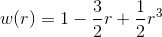• sinusoidal function: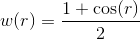• exponential function class
•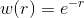•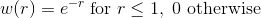•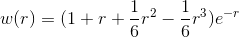• Gaussian functions class:
••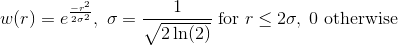•